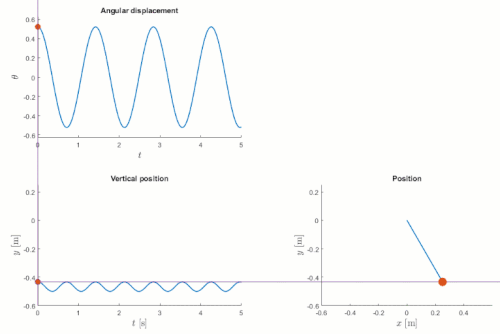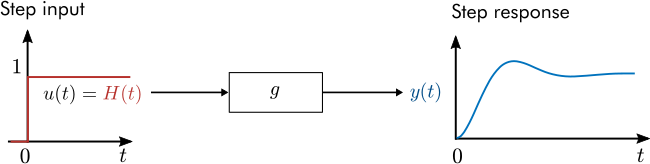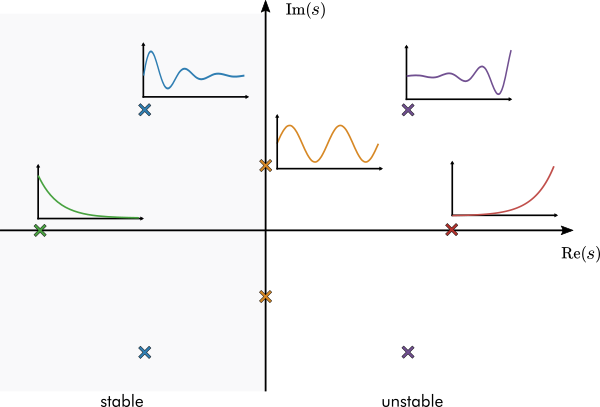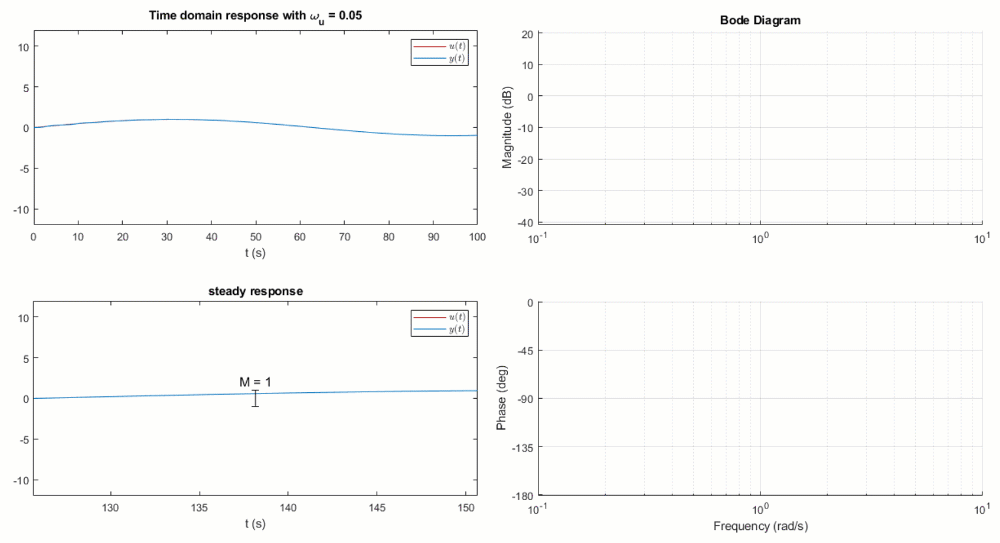## Transfer Function Analysis of Dynamic Systems

버전 1.0.2.0 (2.73 MB) 작성자:
Interactive courseware module that introduces transfer function analysis taught in system dynamics and controls courses.

다운로드 수: 2.7K

업데이트 날짜: 2023/3/13

GitHub에서 호스트

# Transfer Function Analysis of Dynamic SystemsCurriculum Module
Created with R2020b. Compatible with R2020b and later releases.

## Description

This curriculum module contains interactive live scripts and a MATLAB® app that teach transfer function analysis of dynamic systems. In the first script, students learn to derive transfer functions from ODEs and compute impulse, step, and forced responses. In subsequent scripts, students perform pole-zero and frequency domain analyses. Throughout the module, students apply transfer functions to analyze the dynamics of physical and electrical systems. In the final lesson, students perform a frequency domain analysis of an LC filter in a buck converter. A review of Laplace transforms is also included for students seeking a refresher. These lessons can be used as part of a lecture, as activities in an instructional setting, or as interactive assignments to be completed outside of class.

The instructions inside the live scripts will guide you through the exercises and activities. Get started with each live script by running it one section at a time. To stop running the script or a section midway (for example, when an animation is in progress), use the Stop button in the RUN section of the Live Editor tab in the MATLAB Toolstrip.

## Suggested Prework

MATLAB Onramp – a free two-hour introductory tutorial that teaches the essentials of MATLAB.
Introduction to Symbolic Math with MATLAB – a two-hour self-paced introductory course that teaches the basics of symbolic mathematics in MATLAB. Note that this course is only available to users with access to the Online Training Suite.

## Details

`Part0_ConceptReview.mlx, Part0_ConceptReviewSoln.mlx`
An interactive lesson that reviews Laplace transforms.Learning Goals:

• Compute Laplace transforms by hand and using symbolic math
• Describe the properties of the Laplace transform
• Apply Laplace transforms to solve initial value problems
• Recall the definition of a linear time-invariant (LTI) operator

`Part1_TransferFunctionBasics.mlx, Part1_TransferFunctionBasicsSoln.mlx`
An interactive lesson that teaches how to derive transfer functions and compute time responses analytically and in MATLAB.Learning Goals:

• Derive transfer functions by hand
• Derive transfer functions using symbolic math
• Numerically evaluate and plot the impulse, step, and forced responses of a system
• Analytically derive the step and forced responses of a system
• Explain the physical significance of time responses

`Part2_PoleZeroAnalysis.mlx, Part2_PoleZeroAnalysisSoln.mlx`
A live script lesson that teaches pole-zero analysis.Learning Goals:

• Describe how the transfer function of a DC motor is derived
• Identify the poles and zeros of a transfer function
• Assess the stability of an LTI system based on the transfer function poles
• Relate the position of poles in the s-plane to the damping and natural frequency of a system
• Explain how poles of a second-order system relate to its dynamics
• Examine how transfer function zeros affect the dynamics of a system

`Part3_FrequencyDomainAnalysis.mlx, Part3_FrequencyDomainAnalysisSoln.mlx`
An interactive lesson that teaches frequency domain analysis using transfer functions.Learning Goals:

• Explain how a Bode plot is generated
• Use MATLAB to numerically calculate the frequency response of a transfer function
• Discuss how features of the Bode plot relate to characteristics of physical systems
• Describe how to derive a differential equation model for a buck converter with an LC filter
• Apply the Bode plot to analyze an LC filter in a buck converter

`polesApp.mlapp`
A MATLAB app that lets you construct a transfer function by graphically positioning the poles and zeros. You can also compute and plot the impulse and step responses.

## Products

MATLAB, Symbolic Math Toolbox™, Control System Toolbox™

The license for this module is available in the LICENSE.TXT file in this GitHub repository.

## Educator Resources

Have any questions or feedback? Contact the MathWorks online teaching team.

### 인용 양식

Chad Allie (2023). Transfer Function Analysis of Dynamic Systems (https://github.com/MathWorks-Teaching-Resources/Transfer-Function-Analysis-of-Dynamic-Systems/releases/tag/v1.0.2), GitHub. 검색됨 .

##### MATLAB 릴리스 호환 정보
개발 환경: R2020b
R2020b 이상 릴리스와 호환
##### 플랫폼 호환성
Windows macOS Linux
##### 커뮤니티
Distance Learning 커뮤니티에 더 많은 파일이 있습니다

### Community Treasure Hunt

Find the treasures in MATLAB Central and discover how the community can help you!

Start Hunting!

#### tests

버전 게시됨 릴리스 정보
1.0.2.0

See release notes for this release on GitHub: https://github.com/MathWorks-Teaching-Resources/Transfer-Function-Analysis-of-Dynamic-Systems/releases/tag/v1.0.2

1.0.1.0

See release notes for this release on GitHub: https://github.com/MathWorks-Teaching-Resources/Transfer-Function-Analysis-of-Dynamic-Systems/releases/tag/v1.0.1

1.0.0

이 GitHub 애드온의 문제를 보거나 보고하려면 GitHub 리포지토리로 가십시오.
이 GitHub 애드온의 문제를 보거나 보고하려면 GitHub 리포지토리로 가십시오.